Courses

# Test: Solid Mechanics- 2

## 20 Questions MCQ Test Mock Test Series of SSC JE Civil Engineering | Test: Solid Mechanics- 2

Description
This mock test of Test: Solid Mechanics- 2 for SSC helps you for every SSC entrance exam. This contains 20 Multiple Choice Questions for SSC Test: Solid Mechanics- 2 (mcq) to study with solutions a complete question bank. The solved questions answers in this Test: Solid Mechanics- 2 quiz give you a good mix of easy questions and tough questions. SSC students definitely take this Test: Solid Mechanics- 2 exercise for a better result in the exam. You can find other Test: Solid Mechanics- 2 extra questions, long questions & short questions for SSC on EduRev as well by searching above.
QUESTION: 1

Solution:
QUESTION: 2

### The equivalent length of a column of length L having one end fixed and the other end free is

Solution:

Effective length of columns under different end conditions are:

1. For both ends hinged: Le = L

2. One end fixed and another end free: Le = 2L

3. Both ends fixed =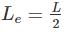4. One end fixed and other is hinges: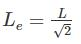QUESTION: 3

### Two bars of different materials are of the same size and are subjected to same tensile forces. If the bars have unit elongations in the ratio of 3 : 8, then the ratio of moduli of elasticity of the two materials is:

Solution:
QUESTION: 4

The radius of Mohr’s circle for two equal and unlike principal stresses of magnitude “p” is:

Solution:

Radius of Mohr’s circle is given by: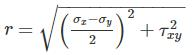At principal plane, σx = σ1, σy = σ2

τxy = 0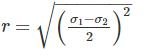σ= p , σ2 = -p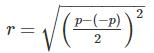r = p

QUESTION: 5

The correct relation between Modulus of elasticity (E), Shear Modulus (G) and Bulk modulus (K) is:

Solution:

Relation between Modulus of elasticity (E), Shear Modulus (G) and Bulk modulus (K) is given by: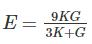Now, rewriting the equation as follows:

3KE + GE = 9KG

3KE – 9KG = - GE

K(3E – 9G) = - GE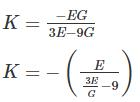QUESTION: 6

A simply supported beam with a gradually varying load from zero at B and w per unit length at A is shown in figure below, the shear force at B is equal to:Solution:

SFy = 0

RA + RB =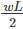Taking moment of all the forces about B = 0.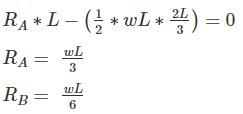QUESTION: 7

When the shear force diagram is a parabolic curve between two points, it indicates that there is a

Solution:

The general relationship between the shear force diagram, bending moment diagram and loading diagram will be:

2. Bending moment diagram is 1higher than shear force diagram.

3. For the uniformly varying load on the beam, the shear force diagram is parabolic in nature.

4. For the uniformly varying load on the beam, the bending moment diagram is also parabolic but is 1o higher than shear force diagram.

QUESTION: 8

The ratio of moment of inertia of circular plate to that of square plate of equal depth is:

Solution:
QUESTION: 9

A circular shaft of 50 mm diameter is required to transmit a torque from one shaft to another. If shear stress is not to exceed 40 Mpa, the safe torque is:

Solution:

Using the relation: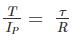Where,

T = Torque applied to the shaft

IP = Polar section modulus

τ = shear stress in the material

R = radius of the shaft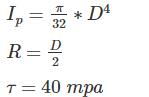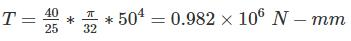= 0.982 kN-m

QUESTION: 10

Maximum Shear strain Energy theory was postulated by:

Solution:

1. Maximum Principal stress theory was postulated by Rankine. It is suitable for brittle materials.

2. Maximum Principal strain theory was postulated by St Venant. This theory is not accurate for brittle and ductile materials both.

3. Maximum Shear stress theory was postulated by Tresca. This theory is suitable for ductile materials. Its results are the safest.

4. Maximum shear strain energy theory was postulated by Von-mises. Its results in case of pure shear are the accurate for ductile materials.

QUESTION: 11

If a shaft turns at 150 rpm under a torque of 1500 Nm, then power transmitted is

Solution: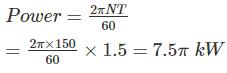QUESTION: 12

If a close coiled helical spring absorbs 30 N-mm of energy while extending by 5mm, its stiffness will be ______.​

Solution: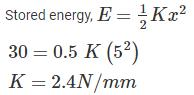QUESTION: 13

A steel bar of 5mm is heated from 5°C to 40°C and is free to expand. The bar will induce

Solution:

When a material undergoes a change in temperature, it either elongates or contracts depending upon whether temperature is increased or decreased of the material. If the elongation or contraction is not restricted, i. e. free then the material does not experience any stress despite the fact that it undergoes a strain.

QUESTION: 14

A bar of uniform thickness t and Length L tapers uniformly from a width of b1 at one end to b2 at the other end. What will be the extension of the bar under an axial pull P?

Solution:
QUESTION: 15

The number of elastic constants for a completely anisotropic elastic material which follows Hooke’s law is

Solution:

Explanation : Non isotropic or anisotropic materials have different properties in different directions. They show non- homogeneous behaviour. The number of elastic constants is 21.

QUESTION: 16

The Poisson’s ratio of a material which has Young’s modulus of 120 GPa, and shear modulus of 50 GPa, is

Solution: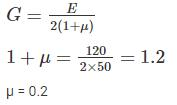QUESTION: 17

The change in the slope between two points in a straight member under flexure is equal to the area of M/EI diagram between those two points. This statement is known as -

Solution:
QUESTION: 18

A simply supported beam is of rectangular section. It carries a uniformly distributed load over the whole span. The deflection at the centre is “y”. If the depth of the beam is doubled, the deflection at centre will be:

Solution:

Deflection of beam is inversely proportional to area moment of inertia of the beam.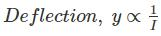Moment of inertia for rectangular section,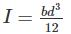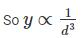Let the final deflection is yfinal when the depth is doubled.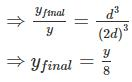QUESTION: 19

The ratio of the moment of resistance of a solid circular shaft of diameter D and a hollow shaft (external diameter D and internal diameter d) is given by

Solution:

As we know,

M ∝ Z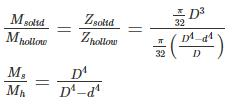QUESTION: 20

Rankine theory is applicable to the ________.

Solution:

For short or long columns Rankine’s Formula is used.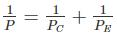Where P is crippling load by Rankine formula;  PC is crushing load; PE is crippling load by Euler’s formula.

For Short column: PE is very large so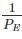will be very small and negligible as compared to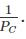so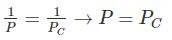For Long column: PE is small so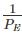will be large as compared to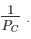Hence the value of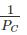can be neglected. So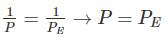Hence the crippling load by Rankine’s formula for long column is approximately equal to the crippling load by Euler’s formula.